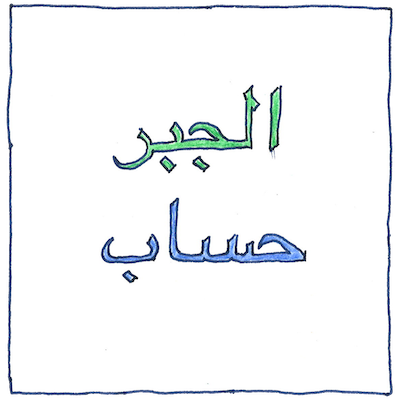# 820

## The book of science

Tom Sharp

 Muḥammad ibn Mūsā al-Khwārizmī mathematics

## Algebra

• Considering only positive numbers,
• al-Khwārizmī divided linear and quadratic equations
• into six basic types and showed how to solve them.
• Although his book was likely a compendium
• of existing knowledge, it stands as the first work
• to feature a strictly algebraic mathematical approach
• so that Latin translations of it in the 12th century
• introduced to the West not only the math but the terms
• algebra and, based on al-Khwārizmī’s name, algorithm.

## Operations

• The al-ğabr operation
• is moving a negative quantity on one side of the equation
• to a positive quantity on the other side.
• This restores negative quantities
• which Islamic mathematicians
• at the time
• did not find useful.
• The al-Muqabala operation
• is subtracting the same positive quantity from both sides.
• This balancing or corresponding act
• reduces the equation to the fewest terms.

## Variable unknown

• Let x be an unknown quantity
• of a variable thing,
• unlike the E in E = mc2.
• When I say x, I mean
• anything that’s consistent,
• not necessarily energy.
• Let x = f(y), where
• f is an arbitrary function
• that depends on a variable y.
• Our function could return an interest,
• the return on a bond,
• or the percentage of profit.
• In fact, it could be
• any useful operation, anything
• that’s smart and practical.
• Now, indeed, we
• are moving to a higher order
• of math and intellect.

Unfamiliarity with the notation can make a person feel as though a mathematical topic is beyond their intellectual powers when it is not.

See also in The book of science:

Readings on wikipedia: